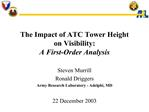# The Impact of ATC Tower Height on Visibility: A First-Order Analysis - PowerPoint PPT PresentationDownload PresentationThe Impact of ATC Tower Height on Visibility: A First-Order Analysis

The Impact of ATC Tower Height on Visibility: A First-Order Analysis
Download Presentation## The Impact of ATC Tower Height on Visibility: A First-Order Analysis

- - - - - - - - - - - - - - - - - - - - - - - - - - - E N D - - - - - - - - - - - - - - - - - - - - - - - - - - -
##### Presentation Transcript

1. The Impact of ATC Tower Height on Visibility: A First-Order Analysis Steven Murrill Ronald Driggers Army Research Laboratory - Adelphi, MD 22 December 2003

3. Analysis Approach Generate probability of discrimination curves using: Standard Target Transfer Probability Function (TTPF)1 Modified Johnson�s discrimination criteria Effective number of spatial cycles on target (aircraft) calculated by scaling the human eye�s Contrast Transfer Function2 (CTFeye) by the atmospheric (optical) turbulence modulation transfer function3 (MTFturb) at each range. A constant contrast transfer value for all ranges. Assess impact of tower height on visibility from the probability of discrimination curves.

4. Contrast Transfer Function (CTF): Human eye

5. Example Turbulence MTF

6. Analysis Assumptions: General: 1) The target (aircraft) size is taken to be the square root of the frontal cross-sectional area of the aircraft (wing span x height). 2) Modified Johnson's criteria is used for the number of cycles required for a 50% probability of success in target discrimination (N50). 3) All observations are made with the unaided eye.

7. Analysis Assumptions (cont�d): General (cont�d): 4) The observer is assumed to be at the specified tower height while all aircraft are taken to be at the ~ 3 ft (1 m) height . 5) ** To account for the impact of turbulence on the downward-slanting optical path, an average/effective refractive-index-structure-parameter scaling factor was calculated. This scaling factor was derived by taking the line integral of the Tatarski height scaling equation over the downward-slanting optical path.

8. Analysis Assumptions (cont�d): Specific: 1) N50 for target detection = 0.75, N50 for target recognition = 3.0, N50 for target identification = 6.0. 2) For calculation of the Contrast Transfer Function (CTF), the square root of the picture area (degrees) (of the "display") is taken to be the FOV of the eye: - FOV of the eye for aircraft DETECTION includes both photopic and scotopic vision: FOV(detection) is taken to be 40 (degrees). - FOV of the eye for aircraft RECOGNITION and IDENTIFICATION is primarily photopic: FOV (recognition, identification) is taken to be 10 (degrees).

9. Analysis Assumptions (cont�d): Specific (cont�d): 3) The target-to-background luminance ratio is taken to equal 2.0. (CT = (Lt - Lb)/(Lt + Lb) = 0.33) 4) Weather conditions include: a hot sunny day at 5 to 6 temporal hour (display luminance (L) = 1E4 cd/meter^2). 5) The atmospheric index structure parameter (Cn2) (@ 1 m above ground) is taken to be 1E-12 (m-2/3)1. 6) The tower heights used in this analysis are: 110, 130, & 150 (ft). 7) The average wavelength of light from the aircraft is 550 (nm).

10. Probability of Detection: Cessna 172

11. Probability of Recognition: Cessna 172

12. Probability of Identification: Cessna 172

13. Probability of Detection: Lear 60

14. Probability of Recognition: Lear 60

15. Probability of Identification: Lear 60

16. Probability of Detection: ConVair 580

17. Probability of Recognition: ConVair 580

18. Probability of Identification: ConVair 580

19. Observations This analysis was performed using worst-case assumptions for most parameters. The calculation of the probability of discrimination curves is based on an average/effective refractive-index-structure-parameter scaling factor which was calculated to account for the impact of turbulence on the downward-slanting optical path. The scaling factor was derived by taking the line integral of the Tatarski height scaling equation over the downward-slanting optical path: A more accurate result would be obtained if an appropriate weighting factor was incorporated into the line integral equation to account for the effect of distance between the point of integration and the observer. This factor could be added in a future program update.

20. Summary The maximum change in visibility (range of discrimination) occurs between the 110 and 150 ft tower heights (as expected) at the 50 % probability-of-detection level. Those maximum changes were calculated to be: Cessna 172: ~ 6.9 % Lear 60: ~ 7.9 % ConVair 580: ~ 8.8 % At 4500 ft (~ 1.4 km), the probabilities of identification for the Cessna 172 are all just below the 90 % level; the maximum change in visibility is < 2 % among the three tower heights analyzed (at this maximum range of interest for this analysis).